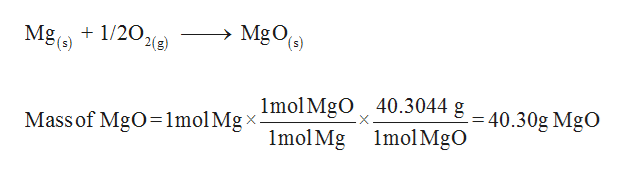Assume that you start with 1 mole(s) of magnesium metal, determine:The number of grams of MgO(s) that would be produced in the following reaction (enter your answer with 4 significant figures):Mg(s) + 1/2O2(g) → MgO(s)  The number of grams of Mg3N2(s) that would be produced in the following reaction (enter your answer with 4 significant figures):Mg(s) + 1/3N2(g) → 1/3Mg3N2(s)

Question

Assume that you start with 1 mole(s) of magnesium metal, determine:

The number of grams of MgO(s) that would be produced in the following reaction (enter your answer with 4 significant figures):

Mg(s) + 1/2O2(g) → MgO(s)

The number of grams of Mg3N2(s) that would be produced in the following reaction (enter your answer with 4 significant figures):

Mg(s) + 1/3N2(g) → 1/3Mg3N2(s)

Step 1

The mass of magnesium oxid...help_outlineImage TranscriptioncloseMgOe) Mg(s) 1/20, 2(g) Mass of MgO=1molMgximolMgO 40.3044 g-40.30g MgO 1mol Mg 1mol MgO fullscreen

Want to see the full answer?

See Solution

Want to see this answer and more?

Our solutions are written by experts, many with advanced degrees, and available 24/7

See Solution
Tagged in

General Chemistry